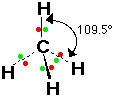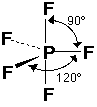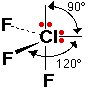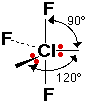# Shapes of Molecules and Ions

$$\newcommand{\vecs}{\overset { \rightharpoonup} {\mathbf{#1}} }$$ $$\newcommand{\vecd}{\overset{-\!-\!\rightharpoonup}{\vphantom{a}\smash {#1}}}$$$$\newcommand{\id}{\mathrm{id}}$$ $$\newcommand{\Span}{\mathrm{span}}$$ $$\newcommand{\kernel}{\mathrm{null}\,}$$ $$\newcommand{\range}{\mathrm{range}\,}$$ $$\newcommand{\RealPart}{\mathrm{Re}}$$ $$\newcommand{\ImaginaryPart}{\mathrm{Im}}$$ $$\newcommand{\Argument}{\mathrm{Arg}}$$ $$\newcommand{\norm}{\| #1 \|}$$ $$\newcommand{\inner}{\langle #1, #2 \rangle}$$ $$\newcommand{\Span}{\mathrm{span}}$$ $$\newcommand{\id}{\mathrm{id}}$$ $$\newcommand{\Span}{\mathrm{span}}$$ $$\newcommand{\kernel}{\mathrm{null}\,}$$ $$\newcommand{\range}{\mathrm{range}\,}$$ $$\newcommand{\RealPart}{\mathrm{Re}}$$ $$\newcommand{\ImaginaryPart}{\mathrm{Im}}$$ $$\newcommand{\Argument}{\mathrm{Arg}}$$ $$\newcommand{\norm}{\| #1 \|}$$ $$\newcommand{\inner}{\langle #1, #2 \rangle}$$ $$\newcommand{\Span}{\mathrm{span}}$$$$\newcommand{\AA}{\unicode[.8,0]{x212B}}$$

This page explains how to work out the shapes of molecules and ions containing only single bonds. The examples on this page are all simple in the sense that they only contain two sorts of atoms joined by single bonds - for example, ammonia only contains a nitrogen atom joined to three hydrogen atoms by single bonds. If you are given a more complicated example, look carefully at the arrangement of the atoms before you start to make sure that there are only single bonds present.

For example, if you had a molecule such as COCl2, you would need to work out its structure, based on the fact that you know that carbon forms 4 covalent bonds, oxygen 2, and chlorine (normally) 1. If you did that, you would find that the carbon is joined to the oxygen by a double bond, and to the two chlorines by single bonds. That means that you couldn't use the techniques on this page, because this page only considers single bonds.

## The electron pair repulsion theory

The shape of a molecule or ion is governed by the arrangement of the electron pairs around the central atom. All you need to do is to work out how many electron pairs there are at the bonding level, and then arrange them to produce the minimum amount of repulsion between them. You have to include both bonding pairs and lone pairs.

First you need to work out how many electrons there are around the central atom:

• Write down the number of electrons in the outer level of the central atom. That will be the same as the Periodic Table group number, except in the case of the noble gases which form compounds, when it will be 8.
• Add one electron for each bond being formed. (This allows for the electrons coming from the other atoms.)
• Allow for any ion charge. For example, if the ion has a 1- charge, add one more electron. For a 1+ charge, deduct an electron.

Now work out how many bonding pairs and lone pairs of electrons there are:

• Divide by 2 to find the total number of electron pairs around the central atom.

• Work out how many of these are bonding pairs, and how many are lone pairs. You know how many bonding pairs there are because you know how many other atoms are joined to the central atom (assuming that only single bonds are formed).

For example, if you have 4 pairs of electrons but only 3 bonds, there must be 1 lone pair as well as the 3 bonding pairs.

Finally, you have to use this information to work out the shape:

• Arrange these electron pairs in space to minimize repulsions. How this is done will become clear in the examples which follow.

### Two electron pairs around the central atom

The only simple case of this is beryllium chloride, BeCl2. The electronegativity difference between beryllium and chlorine is not enough to allow the formation of ions.

Beryllium has 2 outer electrons because it is in group 2. It forms bonds to two chlorines, each of which adds another electron to the outer level of the beryllium. There is no ionic charge to worry about, so there are 4 electrons altogether - 2 pairs. It is forming 2 bonds so there are no lone pairs. The two bonding pairs arrange themselves at 180° to each other, because that's as far apart as they can get. The molecule is described as being linear.## Three electron pairs around the central atom

The simple cases of this would be BF3 or BCl3.

Boron is in group 3, so starts off with 3 electrons. It is forming 3 bonds, adding another 3 electrons. There is no charge, so the total is 6 electrons - in 3 pairs.

Because it is forming 3 bonds there can be no lone pairs. The 3 pairs arrange themselves as far apart as possible. They all lie in one plane at 120° to each other. The arrangement is called trigonal planar.In the diagram, the other electrons on the fluorines have been left out because they are irrelevant.

## Four electron pairs around the central atom

There are lots of examples of this. The simplest is methane, CH4. Carbon is in group 4, and so has 4 outer electrons. It is forming 4 bonds to hydrogens, adding another 4 electrons - 8 altogether, in 4 pairs. Because it is forming 4 bonds, these must all be bonding pairs.

Four electron pairs arrange themselves in space in what is called a tetrahedral arrangement. A tetrahedron is a regular triangularly-based pyramid. The carbon atom would be at the centre and the hydrogens at the four corners. All the bond angles are 109.5°.Note

It is important that you understand the use of various sorts of line to show the 3-dimensional arrangement of the bonds. In diagrams of this sort, an ordinary line represents a bond in the plane of the screen or paper. A dotted line shows a bond going away from you into the screen or paper. A wedge shows a bond coming out towards you.

## Other examples with four electron pairs around the central atom

### Ammonia, NH3

Nitrogen is in group 5 and so has 5 outer electrons. Each of the 3 hydrogens is adding another electron to the nitrogen's outer level, making a total of 8 electrons in 4 pairs. Because the nitrogen is only forming 3 bonds, one of the pairs must be a lone pair. The electron pairs arrange themselves in a tetrahedral fashion as in methane.In this case, an additional factor comes into play. Lone pairs are in orbitals that are shorter and rounder than the orbitals that the bonding pairs occupy. Because of this, there is more repulsion between a lone pair and a bonding pair than there is between two bonding pairs. That forces the bonding pairs together slightly - reducing the bond angle from 109.5° to 107°.

 Greatest repulsionlone pair - lone pairlone pair - bond pair Least repulsionbond pair - bond pair

Be very careful when you describe the shape of ammonia. Although the electron pair arrangement is tetrahedral, when you describe the shape, you only take notice of the atoms. Ammonia is pyramidal - like a pyramid with the three hydrogens at the base and the nitrogen at the top.

### Water, H2OFollowing the same logic as before, you will find that the oxygen has four pairs of electrons, two of which are lone pairs. These will again take up a tetrahedral arrangement. This time the bond angle closes slightly more to 104°, because of the repulsion of the two lone pairs. The shape is not described as tetrahedral, because we only "see" the oxygen and the hydrogens - not the lone pairs. Water is described as bent or V-shaped.

### The ammonium ion, NH4+

The nitrogen has 5 outer electrons, plus another 4 from the four hydrogens - making a total of 9. But take care! This is a positive ion. It has a 1+ charge because it has lost 1 electron. That leaves a total of 8 electrons in the outer level of the nitrogen. There are therefore 4 pairs, all of which are bonding because of the four hydrogens.

The ammonium ion has exactly the same shape as methane, because it has exactly the same electronic arrangement. NH4+ is tetrahedral.Methane and the ammonium ion are said to be isoelectronic. Two species (atoms, molecules or ions) are isoelectronic if they have exactly the same number and arrangement of electrons (including the distinction between bonding pairs and lone pairs).

## The hydroxonium ion, H3O+

Oxygen is in group 6 - so has 6 outer electrons. Add 1 for each hydrogen, giving 9. Take one off for the +1 ion, leaving 8. This gives 4 pairs, 3 of which are bond pairs. The hydroxonium ion is isoelectronic with ammonia, and has an identical shape - pyramidal.## Five electron pairs around the central atom

### Phosphorus(V) fluoride, PF5

Phosphorus (in group 5) contributes 5 electrons, and the five fluorines 5 more, giving 10 electrons in 5 pairs around the central atom. Since the phosphorus is forming five bonds, there can't be any lone pairs. (The argument for phosphorus(V) chloride, PCl5, would be identical.)The 5 electron pairs take up a shape described as a trigonal bipyramid - three of the fluorines are in a plane at 120° to each other; the other two are at right angles to this plane. The trigonal bipyramid therefore has two different bond angles - 120° and 90°.

### A tricky example, ClF3

Chlorine is in group 7 and so has 7 outer electrons. The three fluorines contribute one electron each, making a total of 10 - in 5 pairs. The chlorine is forming three bonds - leaving you with 3 bonding pairs and 2 lone pairs, which will arrange themselves into a trigonal bipyramid. There are actually three different ways in which you could arrange 3 bonding pairs and 2 lone pairs into a trigonal bipyramid. The right arrangement will be the one with the minimum amount of repulsion - and you can't decide that without first drawing all the possibilities.These are the only possible arrangements. Anything else you might think of is simply one of these rotated in space. We need to work out which of these arrangements has the minimum amount of repulsion between the various electron pairs. A new rule applies in cases like this:

Note

If you have more than four electron pairs arranged around the central atom, you can ignore repulsions at angles of greater than 90°.

One of these structures has a fairly obvious large amount of repulsion.In this diagram, two lone pairs are at 90° to each other, whereas in the other two cases they are at more than 90°, and so their repulsions can be ignored. ClF3 certainly won't take up this shape because of the strong lone pair-lone pair repulsion.

To choose between the other two, you need to count up each sort of repulsion.

In the next structure, each lone pair is at 90° to 3 bond pairs, and so each lone pair is responsible for 3 lone pair-bond pair repulsions.Because of the two lone pairs there are therefore 6 lone pair-bond pair repulsions. And that's all. The bond pairs are at an angle of 120° to each other, and their repulsions can be ignored.

Now consider the final structure.Each lone pair is at 90° to 2 bond pairs - the ones above and below the plane. That makes a total of 4 lone pair-bond pair repulsions - compared with 6 of these relatively strong repulsions in the last structure. The other fluorine (the one in the plane) is 120° away, and feels negligible repulsion from the lone pairs.

The bond to the fluorine in the plane is at 90° to the bonds above and below the plane, so there are a total of 2 bond pair-bond pair repulsions. The structure with the minimum amount of repulsion is therefore this last one, because bond pair-bond pair repulsion is less than lone pair-bond pair repulsion. ClF3 is described as T-shaped.

## Six electron pairs around the central atom

### A simple example: SF6

6 electrons in the outer level of the sulphur, plus 1 each from the six fluorines, makes a total of 12 - in 6 pairs. Because the sulfur is forming 6 bonds, these are all bond pairs. They arrange themselves entirely at 90°, in a shape described as octahedral.## Two slightly more difficult examples

### XeF4

Xenon forms a range of compounds, mainly with fluorine or oxygen, and this is a typical one. Xenon has 8 outer electrons, plus 1 from each fluorine - making 12 altogether, in 6 pairs. There will be 4 bonding pairs (because of the four fluorines) and 2 lone pairs.There are two possible structures, but in one of them the lone pairs would be at 90°. Instead, they go opposite each other. XeF4 is described as square planar.

### ClF4-

Chlorine is in group 7 and so has 7 outer electrons. Plus the 4 from the four fluorines. Plus one because it has a 1- charge. That gives a total of 12 electrons in 6 pairs - 4 bond pairs and 2 lone pairs. The shape will be identical with that of XeF4.